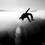# Synthetic Geometry Group-Ambuj Shrivastava's Proposal

These problems are my submission to Xuming's Geometry group.These problems are taken from various sources.
- Q1 In a triangle ABC altitudes are drawn from the vertices A,B & C at points L,M & N respectively.
Prove that : $\frac{AN}{NB}$ * $\frac{BL}{LC}$ * $\frac{CM}{MA}$=1
-Q2 Prove that in any triangle 3 times the sum of squares of the length of sides of triangle is equal to 4 times the sum of squares of the length of medians of the triangle.

-Q3 In a parallelogram ABCD a line segment is drawn from D which meets AB at at F(produced).This line segment DF passes through BC at E(which is the mid point of BC) and through diagonal AC at O
Prove that:OA=$2\times OC$Note by Chaitnya Shrivastava
5 years, 8 months ago

This discussion board is a place to discuss our Daily Challenges and the math and science related to those challenges. Explanations are more than just a solution — they should explain the steps and thinking strategies that you used to obtain the solution. Comments should further the discussion of math and science.

When posting on Brilliant:

• Use the emojis to react to an explanation, whether you're congratulating a job well done , or just really confused .
• Ask specific questions about the challenge or the steps in somebody's explanation. Well-posed questions can add a lot to the discussion, but posting "I don't understand!" doesn't help anyone.
• Try to contribute something new to the discussion, whether it is an extension, generalization or other idea related to the challenge.
• Stay on topic — we're all here to learn more about math and science, not to hear about your favorite get-rich-quick scheme or current world events.

MarkdownAppears as
*italics* or _italics_ italics
**bold** or __bold__ bold
- bulleted- list
• bulleted
• list
1. numbered2. list
1. numbered
2. list
Note: you must add a full line of space before and after lists for them to show up correctly
paragraph 1paragraph 2

paragraph 1

paragraph 2

[example link](https://brilliant.org)example link
> This is a quote
This is a quote
    # I indented these lines
# 4 spaces, and now they show
# up as a code block.

print "hello world"
# I indented these lines
# 4 spaces, and now they show
# up as a code block.

print "hello world"
MathAppears as
Remember to wrap math in $$ ... $$ or $ ... $ to ensure proper formatting.
2 \times 3 $2 \times 3$
2^{34} $2^{34}$
a_{i-1} $a_{i-1}$
\frac{2}{3} $\frac{2}{3}$
\sqrt{2} $\sqrt{2}$
\sum_{i=1}^3 $\sum_{i=1}^3$
\sin \theta $\sin \theta$
\boxed{123} $\boxed{123}$

## Comments

Sort by:

Top Newest

Q1) In any triangle, a line drawn from a vertex to the opposite side is called a cevian. Thus, AL, BM and CN are cevians.

It directly follows from Ceva's theorem that $\dfrac {AN}{BL} \times \dfrac {BL}{BL} \times \dfrac {CM}{MA}=1$

- 5 years, 8 months ago

Log in to reply

Its complete proof would be as follows
triangle ALB similar to triangle CNB by AA criteria . .. $\Rightarrow$ $\frac {LB}{NB}$ = $\frac {AL}{CN}$ $\rightarrow$ 1 triangle ACL similar to triangle BCM by AA criteria . $\Rightarrow$ $\frac {CM}{CL}$ = $\frac {BM}{AL}$ $\rightarrow$ 2 triangle BMA similar to triangle CNA by AA criteria .. $\Rightarrow$ $\frac {NA}{MA}$ = $\frac {CN}{BM}$ $\rightarrow$ 3
Now, On multiplying 1,2 & 3.and rearranging we get
$\frac{AN}{NB}$ * $\frac{BL}{LC}$ * $\frac{CM}{MA}$=1
As required.

- 5 years, 8 months ago

Log in to reply

That's how we prove Ceva's theorem. It's the same thing, although you proved Ceva's theorem. whereas I directly used it

- 5 years, 8 months ago

Log in to reply

Sorry I didn't know about that theorem so i thought it to be a good question but still the proof might be useful.

- 5 years, 8 months ago

Log in to reply

Yeah, It's okay. :)

- 5 years, 8 months ago

Log in to reply

Am i allowed to add more questions to the set because i recently found one.

- 5 years, 8 months ago

Log in to reply

Well, maybe you can add it as a different note for the benefit of everyone.

- 5 years, 8 months ago

Log in to reply

Okay thank you.I have a doubt regarding cevea theorem can you please explain that how can we prove it in case of an ordinary cevian that is when its not an altitude, angle bisector or median.

- 5 years, 8 months ago

Log in to reply

Ceva's theorem states that $\dfrac {AN}{BL} \times \dfrac {BL}{BL} \times \dfrac {CM}{MA}=1$ is true for any three cevians of a triangle, not only angle bisectors, or perpendiculars, or Medians

- 5 years, 8 months ago

Log in to reply

You typoed the theorem.

- 5 years, 8 months ago

Log in to reply

Please change it, my lord.

- 5 years, 8 months ago

Log in to reply

Yeah but I was thinking about its proof!!

- 5 years, 8 months ago

Log in to reply

Q2) From Appolonius' theorem, We have

$AB^2 + AC^2 = 2(AD^2+ \dfrac {BD^2}{4}) \\ AB^2 + BC^2 = 2(BE^2+ \dfrac {AD^2}{4}) \\ AC^2 + BC^2 = 2(CF^2+ \dfrac {AB^2}{4})$

Adding all these, and transposing (I'm sorry for posting the incomplete solution, It's night here.)

$3 (AB^2+BC^2+CA^2)= 4(AD^2+BE^2+CF^2)$

- 5 years, 8 months ago

Log in to reply

Q3

We have $\Delta OAD$ similar to $\Delta OCE$ by the $AAA$ criterion. ($\angle DOA = \angle COE$, $\angle ODA = \angle OEC$, $\angle OAD = \angle OCE$). Furthermore, $2CE = AD$, which implies that $\Delta OAD$ is an enlargenment of $\Delta OCE$ by a factor of 2. This implies that $OA = 2OC$ since they are corresponding sides on the triangles. Therefore, $OA = 2 \times OC$.

- 5 years, 8 months ago

Log in to reply

Nicely explained

- 5 years, 8 months ago

Log in to reply

×

Problem Loading...

Note Loading...

Set Loading...